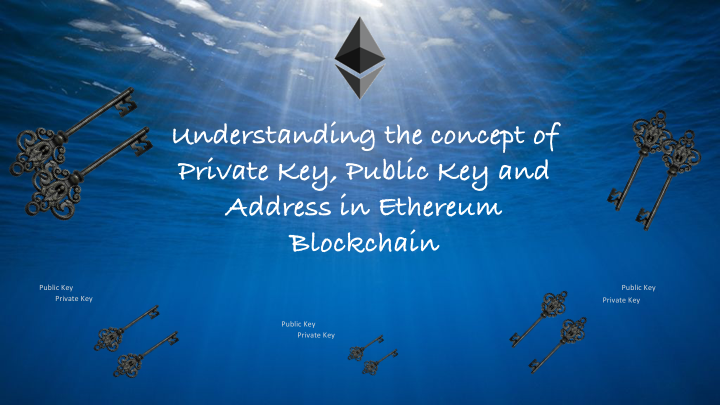### Understanding the concept of Private Key, Public Key and Address in Ethereum Blockchain

Before we get deeper into the concept of Ethereum Blockchain, it is important to understand how Public Key, Private Key and Address are generated. To make this easier for beginners, I am trying to explain the technical concept with an example.

Ethereum key generation is based on elliptical curve ecp256k1. Eliptical curve (EC) is intense mathematics, and there are a lot of great articles on internet covering deep details of elliptical curve. There are standard libraries to generate Ethereum key pair in much safer way. In this article, just for demonstration, I will use elliptical curve Javascript libaray to perform elliptical curve operations.

# Private Key

On EC ecp256k1 , any number between 1 to 2^256-1 is a valid private key. A good library generate a private key with taking sufficient randomness into account. For this exercise we will take "1" as private key which is an acceptable private key because it lies in specified range mentioned above. Ethereum requires private key to be 256 bit long. Here is the sample code for nodejs.

``````var privateKey=Buffer.alloc(32, 0);
privateKey=1;

console.log("PK::"+privateKey.toString('hex'))
``````

This will print private key in hex which is

PK::0000000000000000000000000000000000000000000000000000000000000001

# Public key

Public key is described as follows in yellow paper.

Where pu is the public key, assumed to be a byte array of size 64 (formed from the concatenation of two positive integers each < 2256) and pr is the private key, a byte array of size 32 (or a single positive integer in the aforementioned range).

This is done using group operation of EC cryptography. To derive public key, private key is multiplied by G.Multiplication used to derive public is EC multiplication which is entirely different from normal multiplication for which I am going to use JS library . G is called generator point which is one of the domain parameters of EC cryptography. G has fixed value for ecp256k1, which is recommended by experts. you can read more here.

``````var EC = require('elliptic').ec;
var BN = require('bn.js');
var ec = new EC('secp256k1');

var G = ec.g; // Generator point
var pk = new BN('1'); // private key as big number
var pubPoint=G.mul(pk); // EC multiplication to determine public point

var x = pubPoint.getX().toBuffer(); //32 bit x co-ordinate of public point
var y = pubPoint.getY().toBuffer(); //32 bit y co-ordinate of public point

var publicKey =Buffer.concat([x,y])

console.log("pub key::"+publicKey.toString('hex'))

``````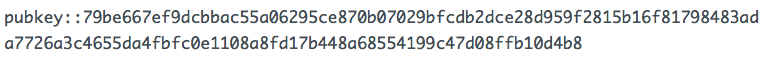Ethereum address is described as follows in yellow paper

For a given private key, pr, the Ethereum address A(pr) (a 160-bit value) to which it corresponds is defined as the right most 160-bits of the Keccak hash of the corresponding ECDSA public key.

To generate Ethereum address, take Keccak-256 hash of public key. Right most 20 bytes is your Ethereum address.

``````var EC = require('elliptic').ec;
var BN = require('bn.js');
var ec = new EC('secp256k1');
const keccak256 = require('js-sha3').keccak256;

var privateKey=Buffer.alloc(32, 0);
privateKey=1;

console.log("PK::"+privateKey.toString('hex'))

var G = ec.g; // Generator point
var pk = new BN('1'); // private key as big number

var pubPoint=G.mul(pk); // EC multiplication to determine public point

var x = pubPoint.getX().toBuffer(); //32 bit x co-ordinate of public point
var y = pubPoint.getY().toBuffer(); //32 bit y co-ordinate of public point

var publicKey =Buffer.concat([x,y])

console.log("public key::"+publicKey.toString('hex'))

const address = keccak256(publicKey) // keccak256 hash of  publicKey

``````

PK::0000000000000000000000000000000000000000000000000000000000000001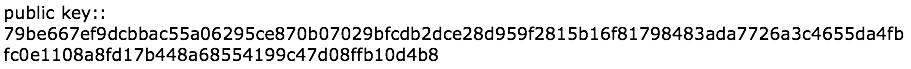This is just an example to understand the concept. Please do not use it to generate real wallet to hold ETH.

If you find this article helpful, you may show your appreciation by sharing it. Also, you may reach me at hello.bitwarrior@gmail.com with your comments, questions or suggestions of any other topic that you would want to be covered at EtherWorld.co.

## Read more articles by BitWarrior and more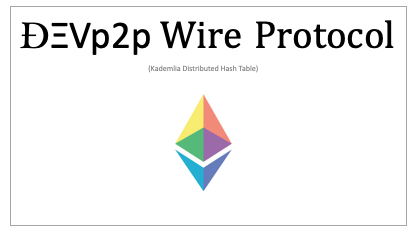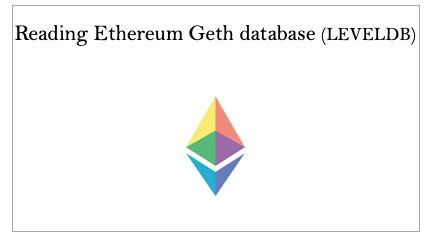EtherWorld's collection of Good Read on Blockchain & Cryptocurrency.

Also try our latest Digital Asset Calculator (DAC) and email your feedback at contact@etherworld.co

____________________________________________________________________________________________________